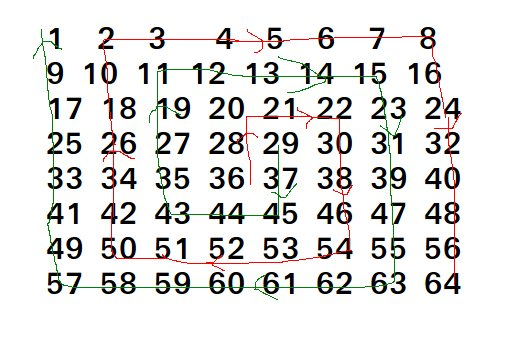# C++ Program to Form coils in a matrix

• Last Updated : 13 Jun, 2022

Given a positive integer n which represents the dimensions of a 4n x 4n matrix with values from 1 to n filled from left to right and top to bottom. Form two coils from the matrix and print the coils.

Examples:

```Input  : n = 1;
Output : Coil 1 : 10 6 2 3 4 8 12 16
Coil 2 : 7 11 15 14 13 9 5 1
Explanation : Matrix is
1  2  3  4
5  6  7  8
9  10 11 12
13 14 15 16

Input  : n = 2;
Output : Coil 1 : 36 28 20 21 22 30 38 46 54
53 52 51 50 42 34 26 18 10
2 3 4 5 6 7 8 16 24 32 40
48 56 64
Coil 2 : 29 37 45 44 43 35 27 19 11 12
13 14 15 23 31 39 47 55 63 62
61 60 59 58 57 49 41 33 25 17
9 1 ```The total elements in the matrix are 16n2. All elements are divided into two coils. Every coil has 8n2 elements. We make two arrays of this size. We first fill elements in coil1 by traversing them in the given order. Once we have filled elements in coil1, we can get elements of other coil2 using formula coil2[i] = 16*n*n + 1 -coil1[i].

## C++

 `// C++ program to print 2 coils of a` `// 4n x 4n matrix.` `#include` `using` `namespace` `std;`   `// Print coils in a matrix of size 4n x 4n ` `void` `printCoils(``int` `n)` `{` `    ``// Number of elements in each coil` `    ``int` `m = 8*n*n;`   `    ``// Let us fill elements in coil 1.` `    ``int` `coil1[m];`   `    ``// First element of coil1` `    ``// 4*n*2*n + 2*n;` `    ``coil1 = 8*n*n + 2*n;` `    ``int` `curr = coil1;`   `    ``int` `nflg = 1, step = 2;`   `    ``// Fill remaining m-1 elements in coil1[]` `    ``int` `index = 1;` `    ``while` `(index < m)` `    ``{` `        ``// Fill elements of current step from` `        ``// down to up` `        ``for` `(``int` `i=0; i= m)` `                ``break``;` `        ``}` `        ``if` `(index >= m)` `            ``break``;`   `        ``// Fill elements of current step from` `        ``// up to down.` `        ``for` `(``int` `i=0; i= m)` `                ``break``;` `        ``}` `        ``nflg = nflg*(-1);` `        ``step += 2;` `    ``}`   `    ``/* get coil2 from coil1 */` `    ``int` `coil2[m];` `    ``for` `(``int` `i=0; i<8*n*n; i++)` `        ``coil2[i] = 16*n*n + 1 -coil1[i];`   `    ``// Print both coils` `    ``cout << ``"Coil 1 : "``;` `    ``for``(``int` `i=0; i<8*n*n; i++)` `        ``cout << coil1[i] << ``" "``;` `    ``cout << "` `Coil 2 : ";` `    ``for` `(``int` `i=0; i<8*n*n; i++)` `        ``cout << coil2[i] << ``" "``;` `}`   `// Driver code` `int` `main()` `{` `    ``int` `n = 1;` `    ``printCoils(n);` `    ``return` `0;` `}`

Output:

```Coil 1 : 10 6 2 3 4 8 12 16
Coil 2 : 7 11 15 14 13 9 5 1 ```

Time Complexity: O(n2), where n represents the given integer.
Auxiliary Space: O(n2), where n represents the given integer.

Please refer complete article on Form coils in a matrix for more details!

My Personal Notes arrow_drop_up
Recommended Articles
Page :